# OWLV2 Online teachin XCthe Deep Blue Compound ( +https://cvg.cengagenow.com/ilm/takeAssignment/takeCovalentActivity.do?locator%=Dassignment-take&takeAssignmentS[References]The deep blue compound Cu(NH3)4SO4 is made by the reaction of copper(II) sulfate and ammonia.CUSO4 (aq) +4 NH, (aq) → Cu(NH3)4SO4 (aq)If you use 27.0 g of CuSO4 and excess NH3, what is the theoretical yield of Cu(NH3),SO, ?g Cu(NH3)4SO4If you isolate 22.0 g of Cu(NH3)4SO4, what is the percent yield of Cu(NH3)4SO4?Submit AnswerTry Another Version8 item attempts remainingShow Hintsearch

Question
22 viewshelp_outlineImage TranscriptioncloseOWLV2 Online teachin X Cthe Deep Blue Compound ( + https://cvg.cengagenow.com/ilm/takeAssignment/takeCovalentActivity.do?locator%=Dassignment-take&takeAssignmentS [References] The deep blue compound Cu(NH3)4SO4 is made by the reaction of copper(II) sulfate and ammonia. CUSO4 (aq) +4 NH, (aq) → Cu(NH3)4SO4 (aq) If you use 27.0 g of CuSO4 and excess NH3, what is the theoretical yield of Cu(NH3),SO, ? g Cu(NH3)4SO4 If you isolate 22.0 g of Cu(NH3)4SO4, what is the percent yield of Cu(NH3)4SO4? Submit Answer Try Another Version 8 item attempts remaining Show Hint search fullscreen
check_circle

Inroduction

The stoichiometry is directly related to the quantitative relationship present between chemicals which are involved in any specific reaction. With the help of stoichiometry, the maximum mass (amount) of any particular limiting reagent can be determined which is equivalent to theoretical yield.

Given Data:

The mass of CuSO4 is 27.0 g.

The actual yield of Cu(NH3)4SO4 is 22.0 g.

Calculation: Part I

The balanced reaction is shown below.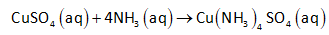It is given that 27 grams of CuSO4 reacts with excess of NH3, this means that CuSO4 is limiting reactant.

The molar mass of CuSO4 is 159.609 g/mol.

The number of moles of CuSO4 can be calculated as follows: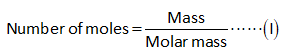Substitute the known values in the above expression.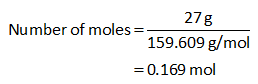According to the balanced equation, apply the stoichiometric rule to determine the moles of Cu(NH3)4SO4 on the basis of limiting reactant CuSO4.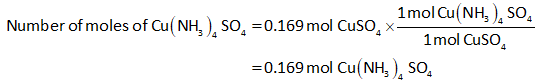The maximum mass of Cu(NH3)4SO4 that can be formed is equivalent to theoretical yield.

The molar mass of Cu(NH3)4SO4 is 227.73 g/mol.

Hence, the mass of Cu(NH3)4SO4 (theoretical yield) is determined by substituting the known values in the expression (I).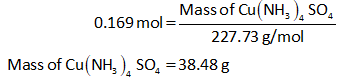Calculation: Part II

The percent yield of Cu(NH3)4SO4 is calculated by using the expression shown below.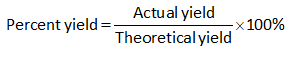Now substitute the known values in the above expression to calculate the percent yield.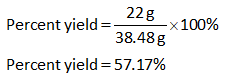...

### Want to see the full answer?

See Solution

#### Want to see this answer and more?

Solutions are written by subject experts who are available 24/7. Questions are typically answered within 1 hour.*

See Solution
*Response times may vary by subject and question.
Tagged in